# Theorem 6.3 (Max-Flow Min-Cut Theorem)

 Theorem 6.3 (Max-Flow Min-Cut Theorem). The maximum value of the flow from a source node s to a sink node t in a capacitated network equals the minimum capacity among all s-t cuts. Similarly, we can show Theorem 6.4 (Augmenting Path Theorem). A flow x* is a maximum flow if and only if the residual network G(x*) contains no augmenting path. We can also show Theorem 6.5 (Integrality Theorem). If all arc capacities are integer, the maximum flow problem has an integer maximum flow. Complexity 0(m) per augmentation max nU (increase flow by 1 each time) 0(nmU) If there are infinite capacity arcs just set uij very large but finite. Drawbacks of the Ford-Fulkerson method 1. Empirically Ford-Fulkerson does well but can construct pathological problems (see book). 2. Irrational capacities cause complications 3. "Forgetfulness" - start labeling over each time - we will discuss improvements. Flows with Lower Bounds lij  0 max v s.t.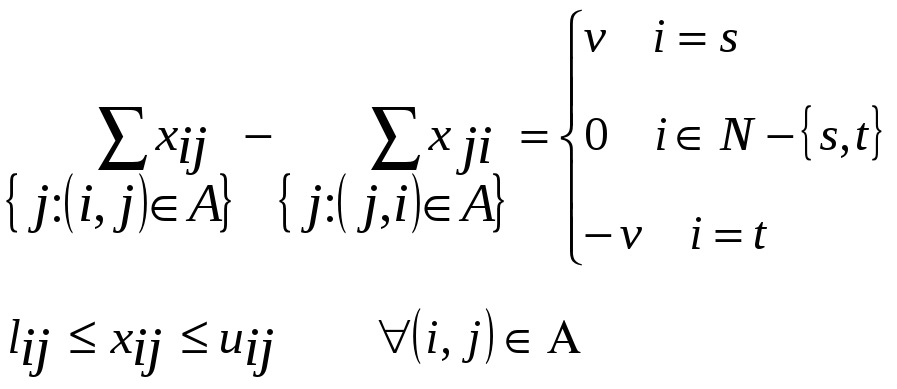Two key steps Show that a feasible flow exists (lij, uij) (6, 9) (4, 5) Infeasible Example 1 2 3 2. Find the maximum flow Consider finding maximum flow first. Assume we have a feasible flow. Now with one modification we can use prior algorithms. Let rij=(uij - xij) + (xji - lji) Since the flow is feasible => lij  xij  uij = > rij  0 Our algorithms work with rij values (not arc flows, capacities, or lower bounds) so we can still use them. One way to construct maximum flows, xij values, from rij values For (i, j) let uij = uij - lij rij = rij xij = xij - lij recall rij = (uij - xij) + (xji - lji) equivalently rij = (uij - xij + xji) similarly rji = (uji - xji + xij) compute x as before xij = max (uij - rij, 0) xji = max (uji - rji, 0) In original variables xij = lij + max (uij - rij - lij, 0) xji = lji + max (uji - rji - lji, 0) Using the modified rij values we will find an optimal solution and can prove theorem 6.10 Theorem 6.10 (Generalized Max-Flow Min-Cut Theorem). If the capacity of an s-t cut [s,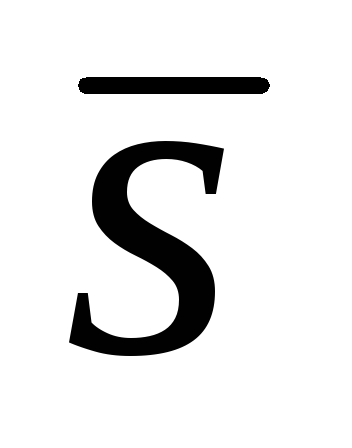] in a network with both lower and upper bounds on arc flows is defined by (6.7), the maximum value of flow from node s to node t equals the minimum capacity among all s-t cuts. (6.7)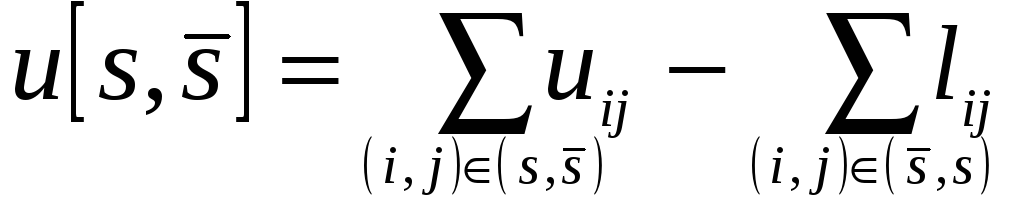How find a feasible flow? First transform to a circulation problem. Add (t, s) with uts =  Find a flow satisfying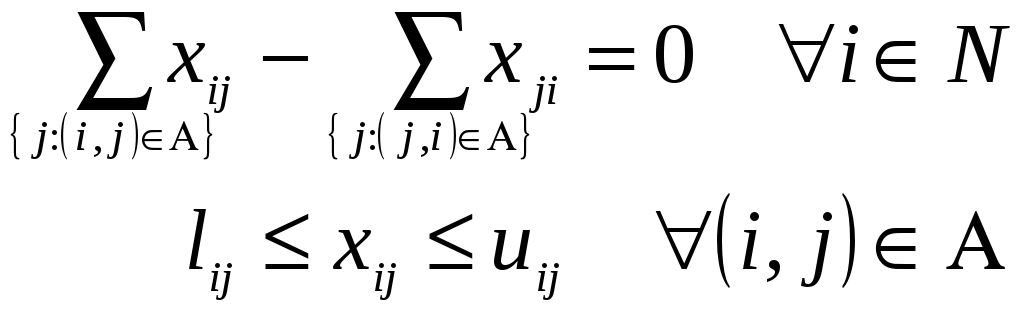(6.11) let xij = xij - lij Now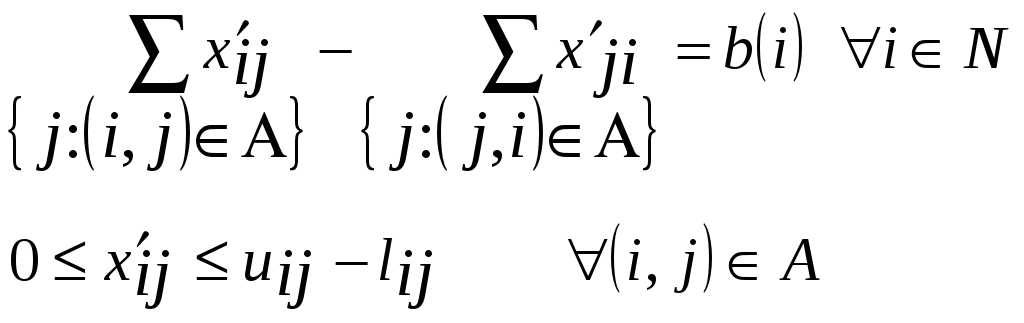(6.2)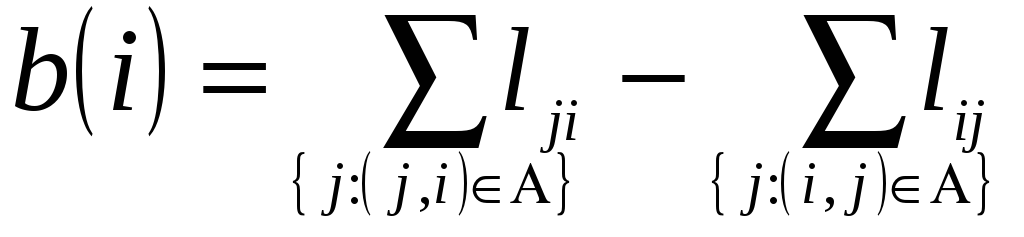Note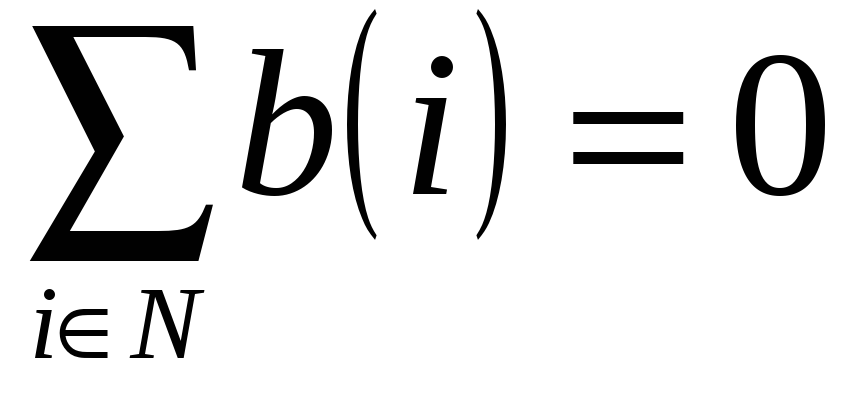Like feasible flow in application 6.1. The maximum flow problem has a feasible solution iff the circulation has a feasible flow. Can show theorem 6.11 Download 1 Mb.Share with your friends: